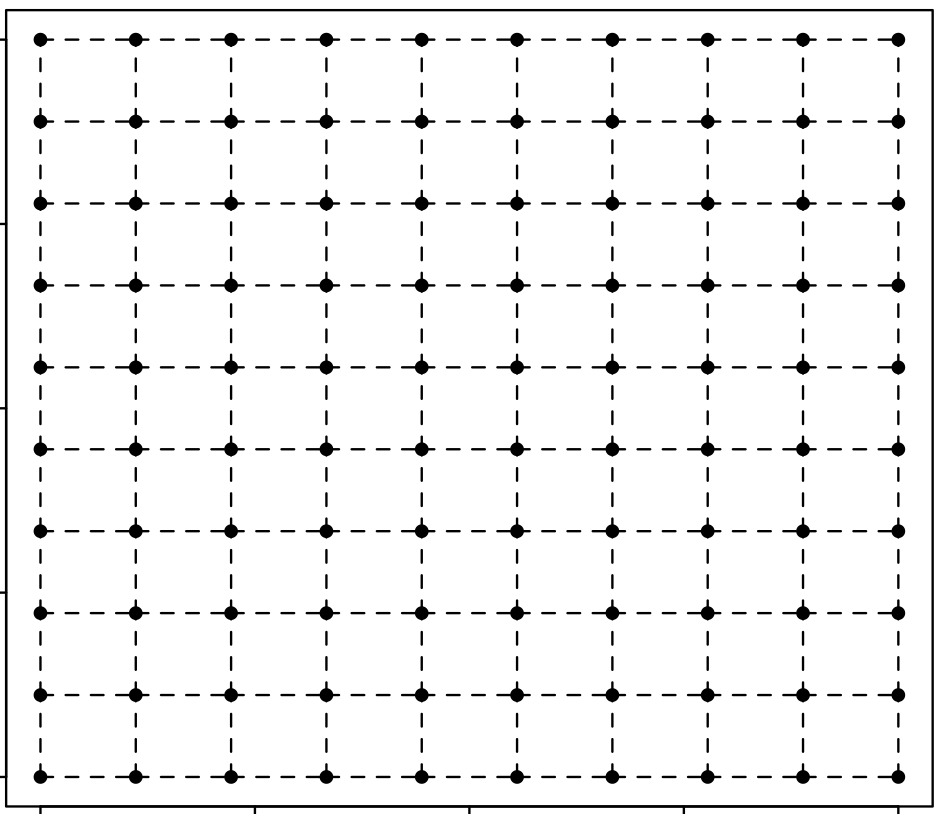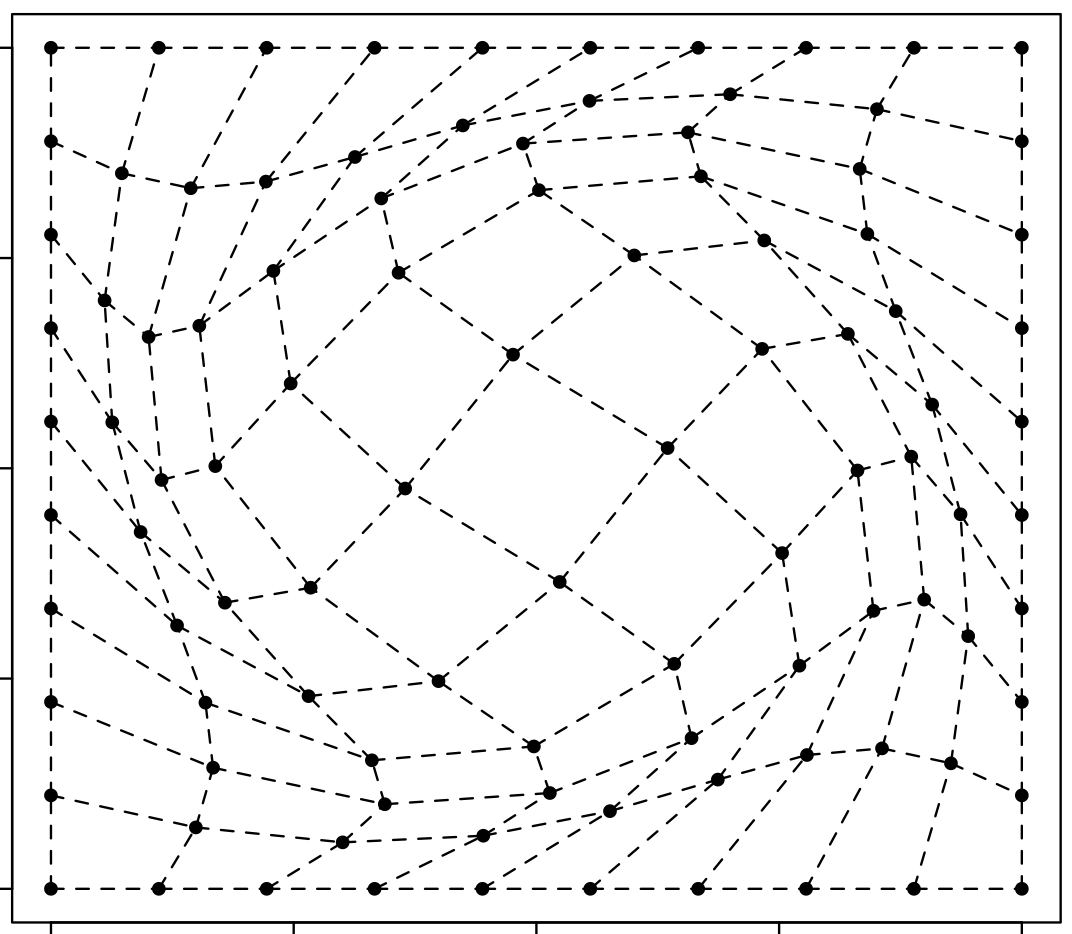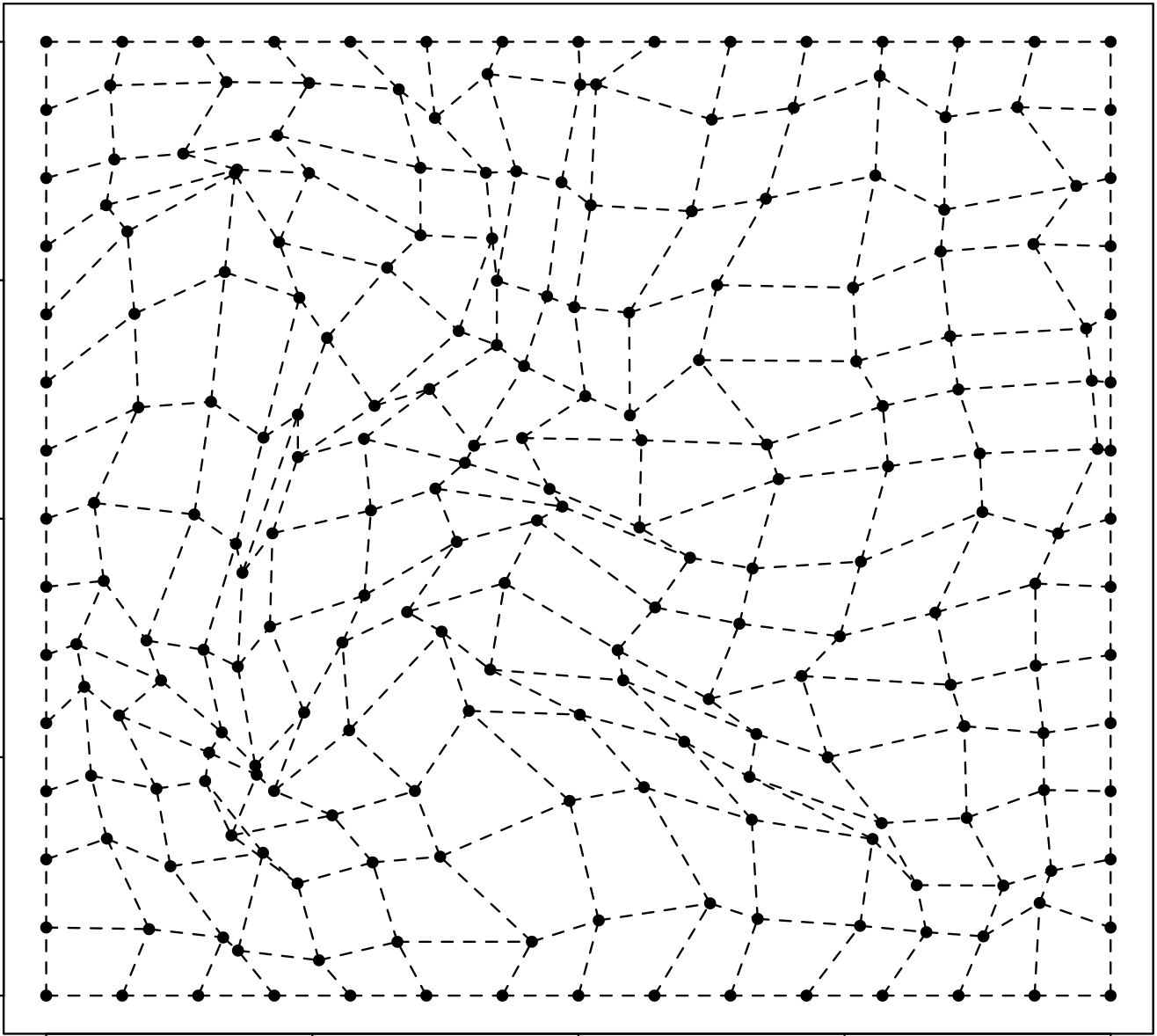The main aim here was to morph space inside a square but such that the transformation preserves some kind of ordering of the points. I wanted to use it to generate some random graphs on a flat surface and introduce spatial deformation to make the graphs more interesting.

I’ve got no idea how to do this - I suppose that the partial derivatives need to be all positive or negative. I believe that I’m after a homeomorphism but I’m out of my depth there… My initial attempt is described below.

I need a transformation $$\psi$$ that maps from points inside a cube $$[-1, 1] \times [-1, 1]$$ to the same cube. The way I’ve tried to do it is by representing the transformation using a multi-output GP (where the outputs are independent). This GP is conditioned such that the border segments of the square are preserved.

$X, Y \sim GP(0, k_{RBF})$ $X, Y : \mathbb R^2 \mapsto \mathbb R$ $\psi : (x, y) \mapsto (X(x, y), Y(x, y))$

All results below start with a uniform grid on a square as the one shown below.## An example of a diffeomorphism

This example is from Wikipedia - I wrote an R implementation based on their (Mathematica?) one here.R Code

## Attempt

Below is some R code that simulates the formalisation above.R Code

Edit: it’d be nice to write up a script such that I can sample from the function conditioned on the gradients (easy to do with GPs as the derivative kernels are known analytically).

## 2020

### Astrophotography

I used to do a fair bit of astrophotography in university - it’s harder to find good skies now living in the city. Here are some of my old pictures. I’ve kept making rookie mistakes (too much ISO, not much exposure time, using a slow lens, bad stacking, …), for that I apologize!

### Probabilistic PCA

I’ve been reading about PPCA, and this post summarizes my understanding of it. I took a lot of this from Pattern Recognition and Machine Learning by Bishop.

### Modelling with Spotify Data

The main objective of this post was just to write about my typical workflow and views rather than come up with a great model. The structure of this data is also outside my immediate domain so I thought it’d be fun to write up a small diary on making a model with it.

# Random Projects

### Morphing with GPs

The main aim here was to morph space inside a square but such that the transformation preserves some kind of ordering of the points. I wanted to use it to generate some random graphs on a flat surface and introduce spatial deformation to make the graphs more interesting.

### SEIR Models

I had a go at a few SEIR models, this is a rough diary of the process.

### Speech Synthesis

The initial aim here was to model speech samples as realizations of a Gaussian process with some appropriate covariance function, by conditioning on the spectrogram. I fit a spectral mixture kernel to segments of audio data and concatenated the segments to obtain the full waveform. Partway into writing efficient sampling code (generating waveforms using the Gaussian process state space representation), I realized that it’s actually quite easy to obtain waveforms if you’ve already got a spectrogram.

### Efficient Gaussian Process Computation

I’ll try to give examples of efficient gaussian process computation here, like the vec trick (Kronecker product trick), efficient toeliptz and circulant matrix computations, RTS smoothing and Kalman filtering using state space representations, and so on.

## 2019

### Gaussian Process Middle C

First of my experiments on audio modeling using Gaussian processes. Here, I construct a GP that, when sampled, plays middle c the way a grand piano would.

Consider: